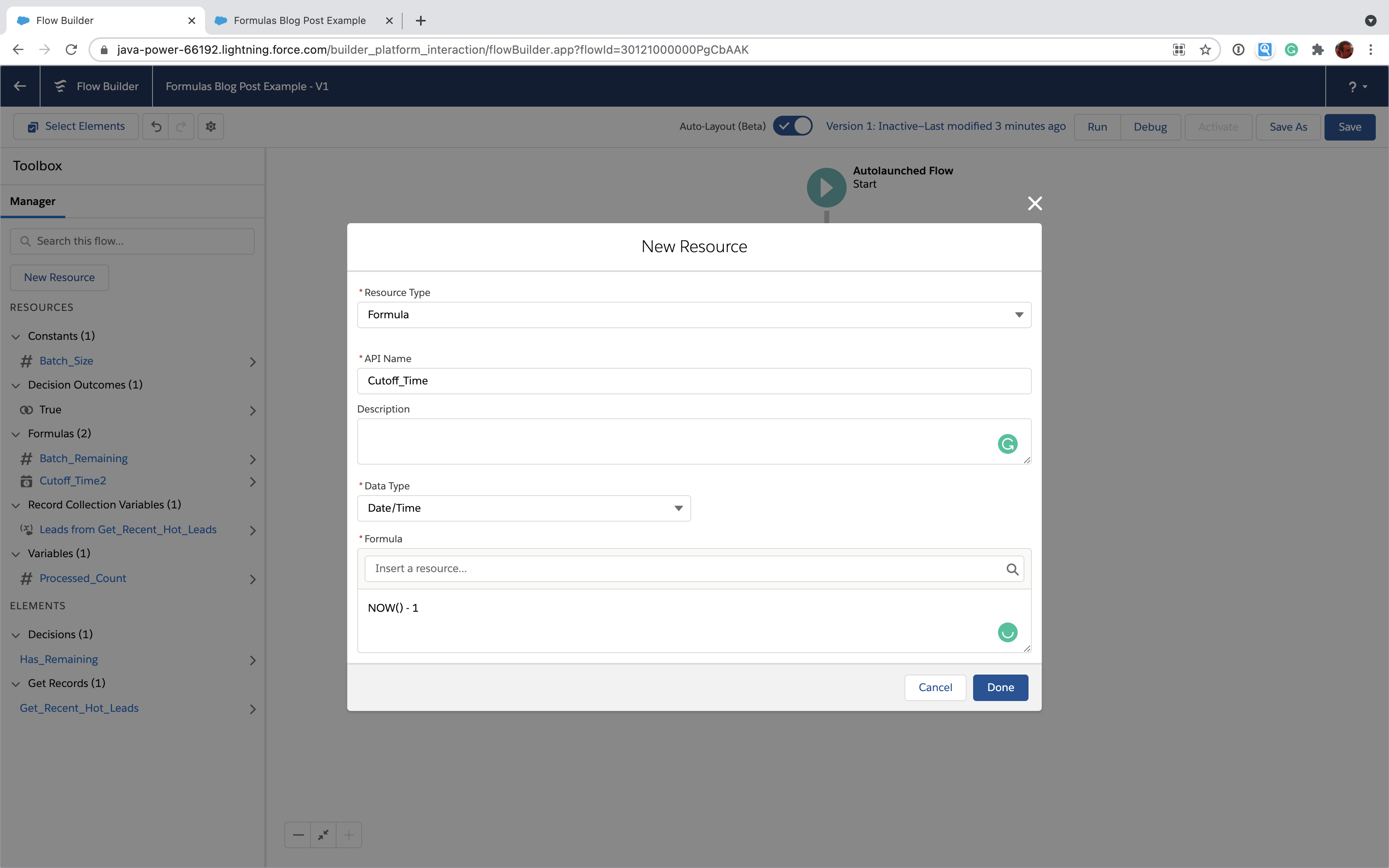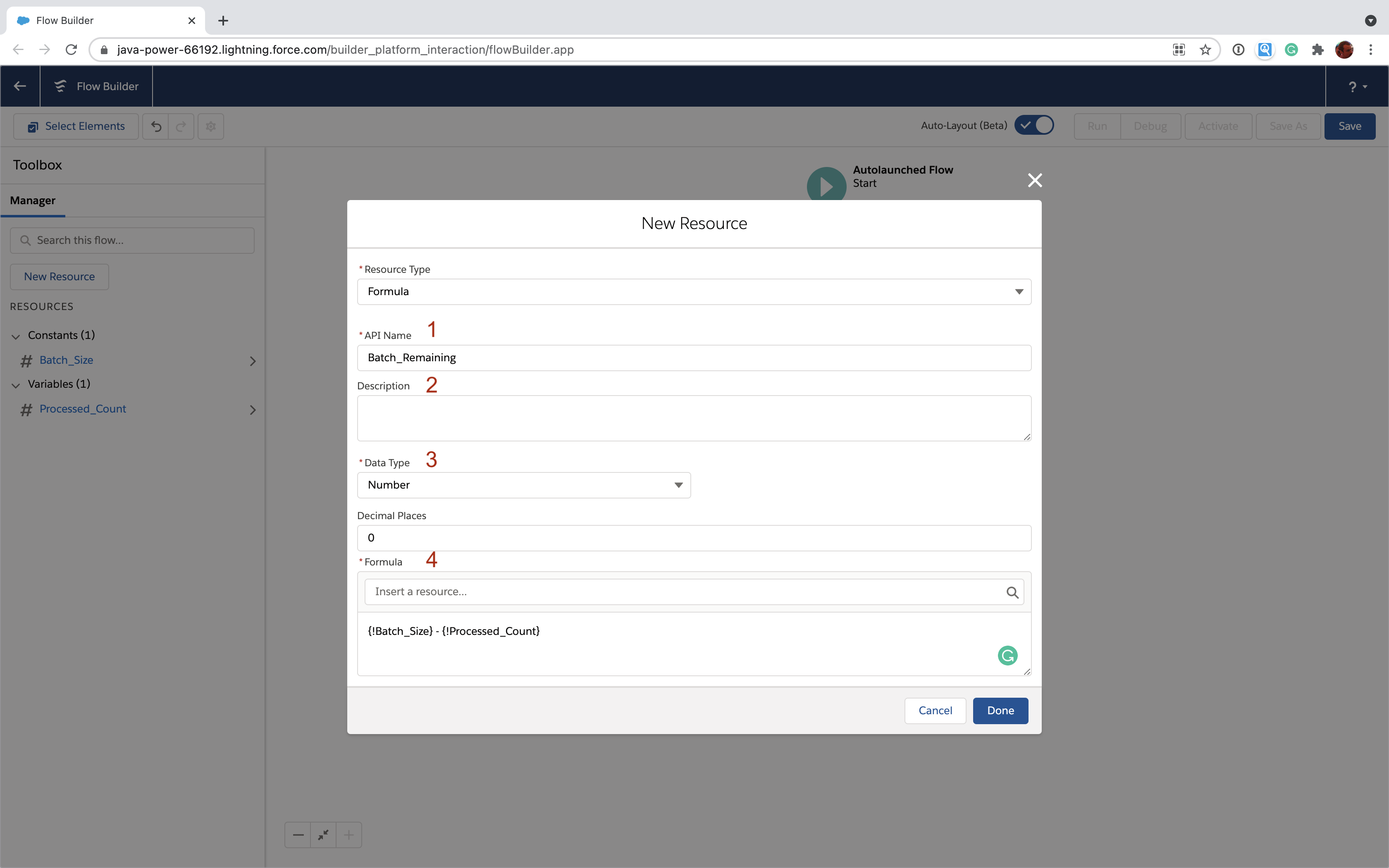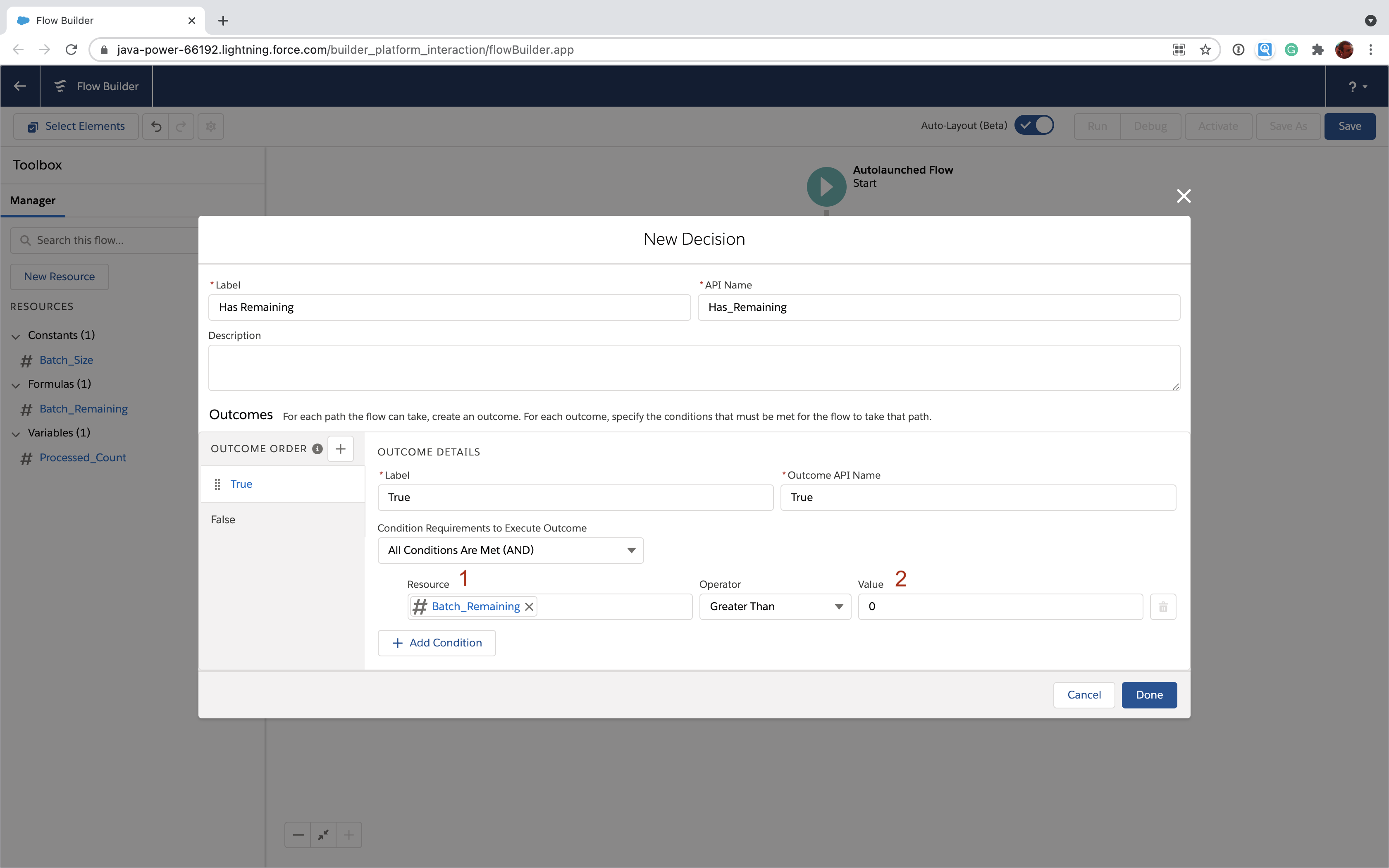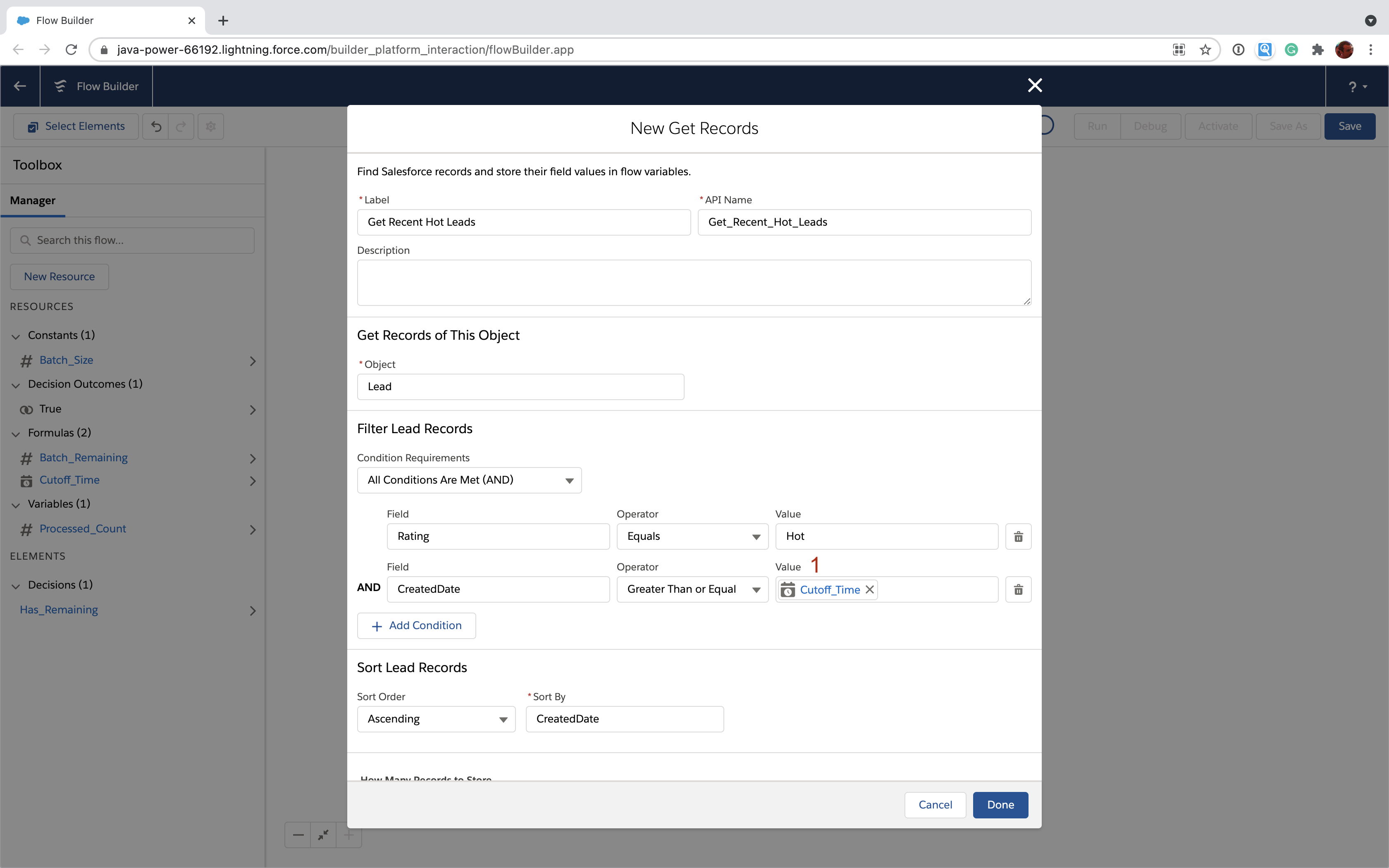04/09/2021

# How to use formulas in Salesforce Flow

This post is part of a technical series to help Salesforce Admins automate business processes using Flow. You may also want to download our free Flow reference guide

You're probably familiar with formula fields in Salesforce. With a little bit of Excel-like code you can create a read-only field that automatically computes a value for you based on other fields. Well, that's exactly how their cousins, formula resources, work in Flow. There are some important differences, though, so it's worth digging a little deeper into how to use formulas in Salesforce Flow.

On the agenda:

• What's a formula in Flow?
• Flow formulas vs custom field formulas
• A useful formula example with date/times

## What's a formula in Flow?

Formulas are resources, just like variables. The big difference is that, unlike variables, you don't directly set a value on the formula resource (i.e. they're "read only"). Whenever you access the value of the resource, it's calculated based on a formula you provide.

Just like custom field formulas, Flow formulas are basically little Excel-like expressions that allow you to use merge fields, operators and functions to compute a value.

To create a formula in the Flow Builder, click "New Resource" in the Toolbox. Here's what you'll see after selecting "Formula" in the "Resource Type" dropdown on the dialog:Like every other resource, a formula has an API Name  that you use to refer to it your Flow and an optional description  that you can use to tell others (or your future self) what the formula is all about.

You also need to specify a data type  to tell Flow what kind of data this formula will produce. Depending what you select for data type, you may need to make a couple of other choices. In this case, we're setting the data type to "Number" so we also tell Flow to ignore any decimal places so we can have a nice, whole number.

Finally, you need to specify the formula itself . In this case, we're merging in two other number resources we defined previously. One, "Batch_Size", is defined as a constant that doesn't change and the second one, Processed_Count, is a variable that we'll change as we process data in the Flow (probably in a loop).

Now you can reference "Batch_Remaining" anywhere in your Flow and it will tell you the current number of items left to process in your batch without having to do any more work. It all happens in the formula using the current values of "Batch_Size" (which never changes) and "Processed_Count" (which does change).

Here's how it would look to use this formula in your flow to make a decision about which steps to take next:You're specifying the formula as your resource  and then testing to see if it's greater than 0  to determine whether you have things remaining in our batch. Pretty neat!

## Flow formulas vs custom field formulas

The first difference between Flow formulas and the formulas you use in custom fields has to do with merge fields. In Flow formulas your merge fields are the other resources (like variables) you create and the various Flow global variables. In custom fields, your merge fields reference other fields on the object.

Flow formulas do handle certain data types differently from custom fields. Here are those differences:

 Data Type Flow Formula Custom Field Formula Checkbox / Boolean Yes (called Checkbox) Yes (called Boolean) Currency Yes Yes Date Yes Yes Date/Time Yes Yes Number Yes Yes Percent Yes No (but you can compute it) Text Yes Yes Time Yes No

Finally, if you're a hardcore formula user, you should know find that not every function you're used to is supported in Flow. Most of the unsupported functions deal with the record changes (e.g. ISNEW, ISCHANGED, PRIORVALUE). Flow provides other ways of dealing with many of those kinds of issues in record-triggered Flows. For more information on all the nuances, check out Salesforce's "considerations" for Flow formulas.

## A useful example

A common use case we've seen for formulas is to include a relative date/time in your Flow. This is useful if you want your Flow to operate on records that have been changed or created recently. Here's a Get Records element with an example of that kind of query.Notice that we're using a resource called "Cutoff_Time" . This is a formula resource that's set to 24 hours in the past. This lets us find only Leads with a "Hot" rating that have been created in the last 24 hours.

Here's how you create the "Cutoff_Time" formula:That's all there is to it. Just use the NOW() formula function and subtract 1. That will subtract a day (24 hours) from the current date and time whenever you run the Flow. This is very useful in Scheduled Flows when you want to use Get Records to fetch a batch of items to process.

## Where to go from here

Flow formulas serve many of the same use cases that custom field formulas do. However, the biggest benefit in Flow is that you can usually use a formula to save yourself steps in the Flow design itself (usually by removing Assignment elements). This can make your Flows easier to follow. So, if you find yourself doing some tricky math or logic with Flow elements operating on several resources, consider trying to transition that to a formula.

You may also want to download our free quick reference guide for Flow

Happy Flowing.

## See it in action## Free Salesforce Flow quick reference

Get our free Flow guide! Keep a copy around for when you need a quick refresher on data and resource types, logic, naming conventions, and so much more.